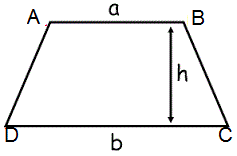# Find Area of Trapezoid

Enter the base and heignt:

 Base a : Base b : Height :

 Area of Trapezoid =

Calculator to find the area of a trapezoid, take the sum of its both given bases, multiply the sum by the given height of the trapezoid, and then divide the result by 2.

The formula to find Area of Trapezoid as follows:

Area = height (base1 + base2)/2## Properties of Trapezium

1. Diagonals intersect each other.

2. The sum of the internal angles is 360°.

3. The two angles are supplementary to each other and their sum is equal to 180°.

4. Exactly one pair of opposite sides are parallel, other than isosceles trapezium, trapezium has non-parallel sides unequal.

5. The line that joins the mid-point of the non-parallel sides is always parallel to the bases of the trapezium.

6. Mid-segment = (AB + CD)/2

7. Trapezium are of three types as : Scalene Trapezium, Isosceles Trapezium and Right Trapezium.

### Comparison between Trapezium and Rectangle

TrapeziumRectangle
All angles are equal
Opposite angles are equal
Opposite sides are parallel   Opposite sides are parallel
Sum of two adjacent angles is 180
Bisect each other